Next: Constants Up: List of Symbols Previous: Notation

# Physical Quantities

 Symbol Unit Description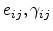1 Engineering strain component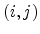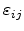1 Component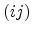of the strain tensor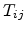GPa Componentof the stress tensor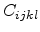GPa Component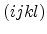of the elastic stiffness tensor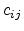GPa Componentof the contracted stiffness tensor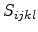GPa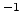Componentof the elastic compliance tensor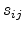GPaComponentof the contracted compliance tensor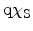eV Electron affinity of the semiconductor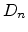msElectron diffusion coefficient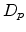msHole diffusion coefficient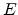VmElectric field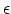eV Energy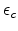eV Conduction band edge energy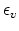eV Valence band edge energyeV Band gap energy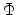V Potential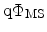eV Work function difference between metal and semiconductor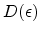m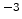eVDensity of states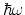eV Phonon energy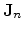Am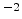Electron current density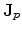AmHole current densitymWave number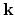mWave number vector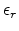1 Relative dielectric permittivity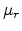1 Relative magnetic permeability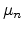mVsElectron mobility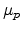mVsHole mobility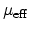mVsEffective electron mobility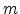kg Mass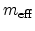kg Carrier effective mass in the semiconductor

 Symbol Unit Description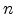mElectron concentration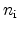mIntrinsic concentration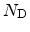mConcentration of donors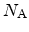mConcentration of acceptors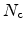mEffective density of states of the conduction band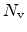mEffective density of states of the valence band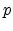mHole concentration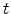s Time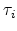s Momentum relaxation time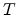K TemperaturemsVelocity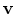msVelocity vector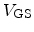V Gate-source voltage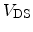V Drain-source voltageNext: Constants Up: List of Symbols Previous: Notation

S. Dhar: Analytical Mobility Modeling for Strained Silicon-Based Devices